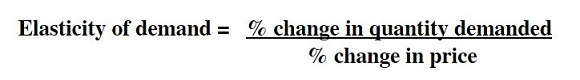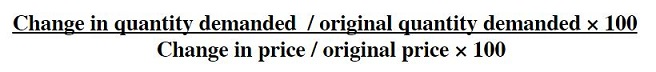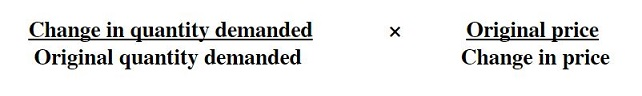# Price Elasticity of Demand.### ECONOMICS.

If the price of a product falls, by how much will demand increase ? By a small amount or a large amount ?

This question is a very important question in economics. The magnitude by which demand (or supply) changes when price changes is very significant. If you were a business executive, for example, you would want to have an idea of how much demand for your product will increase by, if you decided to lower it’s price. Alternatively, if you were a government official and you wanted to place a tax on cigarettes, you would want to know how much tax revenue you would earn by doing this. Understanding elasticity of demand of the product would give you an idea.

The elasticity of demand measures the amount by which demand changes when the price of a product changes  (the ‘sensitivity’ of demand). If the change in price was very small, but the change in quantity demanded was large, then we say that the demand for the product is very elastic (very sensitive). If the opposite were true (the change in price was very large but the change in quantity demanded was small) then we say the demand is inelastic (insensitive).

Elasticity of demand is measured using the following formula:-If the answer to the above equation was <1 then demand is said to be inelastic.

If the answer exactly equaled 1, then elasticity of demand is said to be unitary.

If the answer were >1, then elasticity of demand is elastic.

For example, if in the above equation the change in quantity demanded was 20%, and the change in price was 10%, then demand would be elastic. (20% divided by 10% equals 2, which is greater than one ).

Working out the ‘percentage change’ in something can sometimes be difficult. Another way of formulating the above equation is to break it down even more. To work out the percentage change in a variable you use the following formula:-

Change in variable/original value of variable × 100

Substituting this into the above equation, you get:-Another way of formulating the above equation is to write it like this:-Use what ever method of calculating elasticity of demand you feel most comfortable with. As long as you use the formulas correctly, you will always come up with the correct answer !

Note.

Technically, all formulas for calculating elasticity of demand should have a minus sign in their answer. This is because changes in demand and changes in price always move in opposite directions. If the price of a product falls, then quantity demanded increases (change in price is negative, change in quantity demanded is positive). In practice, economists usually don’t bother to put in the minus sign.

##### Exercises.

Do these exercises to practice the PED calculation. Work out the elasticity of demand for the following examples:-

1) The price of apples rises from \$4 to \$5. Quantity demanded falls from 340 kg per week to 300.

2) The price of cinema tickets rises from \$11 per seat to \$14. Demand falls from 2000 seats to 1800 per week.

3) The price of copper rises from \$3400 per tonne to \$4800. Demand falls from 800 tonnes per week to 200 tonnes.

4) Demand has risen from 33,500 units to 45,000 units because prices fell from \$3.2 to \$1.6.

5) The price of fuel fell from \$ 6 to \$4 per litre. Demand rose from 12,000 litres to 16,000 litres.

6) What do you think determines the elasticity of demand of a product ? Why are some products more elastic than others ?